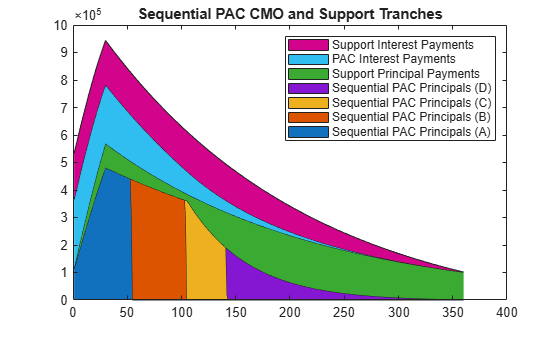# Create PAC and Sequential CMO

This example shows how to use an underlying mortgage-backed security (MBS) pool for a 30-year fixed-rate mortgage of 6% to define a PAC bond, and then define a sequential CMO from the PAC bond. Analyze the CMO by comparing the CMO spread to a zero-rate curve for a 30-year Treasury bond and then calculate the weighted-average life (WAL) for the PAC bond.

### Step 1. Define the underlying mortgage pool.

```principal = 100000000; grossrate = 0.06; coupon = 0.05; originalTerm = 360; termRemaining = 360; speed = 100; delay = 14; Settle = datenum('1-Jan-2011'); IssueDate = datenum('1-Jan-2011'); Maturity = addtodate(IssueDate, 360, 'month');```

### Step 2. Calculate underlying pool cash flow.

```[CFlowAmounts, CFlowDates, ~, ~, ~, UnitPrincipal, UnitInterest, ... UnitPrepayment] = mbscfamounts(Settle, Maturity, IssueDate, grossrate, ... coupon, delay, speed, []);```

### Step 3. Calculate prepayments.

```principalPayments = UnitPrincipal * principal; netInterest = UnitInterest * principal; prepayments = UnitPrepayment * principal; dates = CFlowDates' + delay;```

### Step 4. Generate a plot for underlying MBS payments.

```area([principalPayments'+prepayments', netInterest']) title('Underlying MBS Payments'); legend('Principal Payments (incl. Prepayments)', 'Interest Payments')```### Step 5. Calculate the PAC schedule.

```pacSpeed = [80 300]; [balanceSchedule, pacInitBalance] = ... cmosched(principal, grossrate, originalTerm, termRemaining, ... pacSpeed, []);```

### Step 6. Generate a plot for the PAC principal balance schedule.

```figure; area([pacInitBalance'; balanceSchedule']) title('PAC Principal Balance Schedule'); legend('Principal Balance Schedule');```### Step 7. Calculate PAC cash flow.

```pacTranchePrincipals = [pacInitBalance; principal-pacInitBalance]; pacTrancheCoupons = [0.05; 0.05]; [pacBalances, pacPrincipals, pacInterests] = ... cmoschedcf(principalPayments+prepayments, ... pacTranchePrincipals, pacTrancheCoupons, balanceSchedule);```

### Step 8. Generate a plot for the PAC CMO tranches.

Generate a plot for the PAC CMO tranches:

```figure; area([pacPrincipals' pacInterests']); title('PAC CMO (PAC and Support Tranches)'); legend('PAC Principal Payments', 'Support Principal Payments', ... 'PAC Interest Payments', 'Support Interest Payments');```### Step 9. Create sequential CMO from the PAC bond.

CMO tranches, A, B, C, and D

```seqTranchePrincipals = ... [20000000; 20000000; 10000000; pacInitBalance-50000000]; seqTrancheCoupons = [0.05; 0.05; 0.05; 0.05];```

### Step 10. Calculate cash flows for each tranche.

```[seqBalances, seqPrincipals, seqInterests] = ... cmoseqcf(pacPrincipals(1, :), seqTranchePrincipals, ... seqTrancheCoupons, false);```

### Step 11. Generate a plot for the sequential PAC CMO.

Generate a plot for the sequential PAC CMO:

```figure area([seqPrincipals' pacPrincipals(2, :)' pacInterests']); title('Sequential PAC CMO and Support Tranches'); legend('Sequential PAC Principals (A)', 'Sequential PAC Principals (B)', ... 'Sequential PAC Principals (C)', 'Sequential PAC Principals (D)', ... 'Support Principal Payments', 'PAC Interest Payments', ... 'Support Interest Payments');```### Step 12. Create the discount curve.

```CurveSettle = datenum('1-Jan-2011'); ZeroRates = [0.01 0.03 0.10 0.19 0.45 0.81 1.76 2.50 3.18 4.09 4.38]'/100; CurveTimes = [1/12 3/12 6/12 1 2 3 5 7 10 20 30]'; CurveDates = daysadd(CurveSettle, 360 * CurveTimes, 1); zeroCurve = intenvset('Rates', ZeroRates, 'StartDates', CurveSettle, ... 'EndDates', CurveDates);```

### Step 13. Price the CMO cash flows.

The cash flow for the sequential PAC principal A tranche is calculated using the cash flow functions `cfbyzero`, `cfyield`, `cfprice`, and `cfspread`.

```cflows = seqPrincipals(1, :)+seqInterests(1, :); cfdates = dates(2:end)'; price1 = cfbyzero(zeroCurve, cflows, cfdates, Settle, 4)```
```price1 = 2.2109e+07 ```
`yield = cfyield(cflows, cfdates, price1, Settle, 'Basis', 4)`
```yield = 0.0090 ```
`price2 = cfprice(cflows, cfdates, yield, Settle, 'Basis', 4)`
```price2 = 2.2109e+07 ```
`spread = cfspread(zeroCurve, price2, cflows, cfdates, Settle, 'Basis', 4)`
```spread = 5.5084e-12 ```
`WAL = sum(cflows .* yearfrac(Settle, cfdates, 4)) / sum(cflows)`
```WAL = 2.5408 ```

The weighted average life (WAL) for the sequential PAC principal A tranche is `2.54` years.